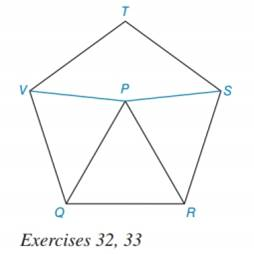Chapter 7.3, Problem 32EElementary Geometry For College St...

7th Edition
Alexander + 2 others
ISBN: 9781337614085

Solutions

Chapter
SectionElementary Geometry For College St...

7th Edition
Alexander + 2 others
ISBN: 9781337614085
Textbook Problem

Given that RSTVQ is a regular pentagon and Δ PQR is equilateral in the figure shown, determine a) the type of triangle represented by Δ VPQ. b) the type of quadrilateral represented by TVPS.To determine

a) To find:

The type of triangle represented by ΔVPQ.

Explanation

It is given that ΔPQR is an equilateral triangle.

An equilateral triangle has all sides angle are congruent.

Therefore, PQ¯QR¯RP¯

All radii of pentagon RSTVQ are congruent.

Therefore, PQ¯VP¯

All sides of a regular pentagon are congruent.

QR¯VQ¯

Substitute PQ¯V

To determine

b) To find:

The type of quadrilateral represented by TVPS.

Still sussing out bartleby?

Check out a sample textbook solution.

See a sample solution

The Solution to Your Study Problems

Bartleby provides explanations to thousands of textbook problems written by our experts, many with advanced degrees!

Get Started